# CBSE Class 10 Physics Electricity Assignment Set B

Read and download free pdf of CBSE Class 10 Physics Electricity Assignment Set B. Get printable school Assignments for Class 10 Physics. Standard 10 students should practise questions and answers given here for Physics in Grade 10 which will help them to strengthen their understanding of all important topics. Students should also download free pdf of Printable Worksheets for Class 10 Physics prepared as per the latest books and syllabus issued by NCERT, CBSE, KVS and do problems daily to score better marks in tests and examinations

## Electricity Class 10 Physics Assignment Pdf

Class 10 Physics students should refer to the following printable assignment in Pdf for Electricity in standard 10. This test paper with questions and answers for Grade 10 Physics will be very useful for exams and help you to score good marks

### Class 10 Physics Assignment for Electricity

Question : The given diagram shows the milliammeter reading connected in a circuit :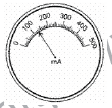The value of current flowing in the circuit is
a. 103 mA
b. 160 mA
c. 100.3 mA
d. 130 mA
Explanation: Least count = 5000mA/50 = 10 mA
No. of divisions = 13

Question : Match the following with the correct response:
(1) Electric current           (A) Ampere
(2) Resistance                 (B) Volt
(3) Potential difference     (C) Ohm
(4) Resistivity                  (D) Ohm-m
a. 1-A, 2-C, 3-B, 4-D
b. 1-D, 2-A, 3-C, 4-B
c. 1-B, 2-D, 3-A, 4-C
d. 1-C, 2-B, 3-D, 4-A
Explanation: The SI unit of electric current is ampere (symbol A). It is named after the French scientist - Andre Marie Ampere. The ohm (symbol Ω) is the SI unit of electrical resistance, named after German physicist - Georg Simon Ohm.
The SI unit of potential difference is volt (symbol V), named after the Italian physicist - Alessandro Volta. The SI unit of resistivity is ohm-metre (symbol Ω- m).

Question : Three bulbs of 100 W, 200 W and 60 W are connected in series to the main supply of 220 V. The current will be:
A. Equal in 100 W and 200 W.
B. Equal in 200 W and 60 W.
C. Different in all bulbs.
D. None of the above
a. A and B
b. B and D
c. A, B and C
d. A and C

Explanation: The current will be same in all the bulbs. In a series combination of resistors, the same current flows through each resistor. The current is the same in every part of the circuit.

Question : Which of the following charges is possible?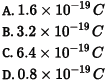a. A, B and C
b. All of these
c. B and C
d. A and C
Explanation: Charges given in A, B and C are possible. 1.6 x 10-19C is the amount of charge on a proton or an electron. This is the minimum charge that any particle will have.

Question : The given figure shows three resistorsFind the combined resistance.
Let the three resistors are R1,R2 and R3. Here R1,R2  are parallel to each other and R3 is in series with them then equivalent resistance can be obtained by the given formula:Question : Find the minimum resistance that can be made using five resistors each of .
For getting minimum resistance R we can connect five resistors in parallel Combination .We know that :
R = 1/5 , n = 5
Req = R/n = 1/5 /5   = 1/25 Ω

Question : Out of the two wires P and Q shown below which one has greater resistance? Justify it.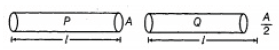Answer :  We know that resistance and cross-section area are inversly proportional to each other. So less area of cross section means more resistance .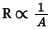[since lengths are same]

So, out of two, wire Q has greater resistance.

Question : Nichrome is used to make the element of electric heater. Why?
Answer :  Nichrome is used to make the element of an electric heater because nicrome is an alloy which has high resistivity and high melting point. That's why nicrome is used to make the element of heater.

Question : An electric iron of resistance 20 Ω takes a current of 5A. Calculate the heat developed in 30s.
Answer :  R = 20 Ω; I = 5A; t = 30 s.
H = I2Rt = (5)2 (20) (30)
H = 15,000 J

Question : A metallic wire of resistance R is cut into ten parts of equal length. Two pieces each are joined in series and then five such combinations are joined in parallel. What will be the effective resistance of the combination? (3)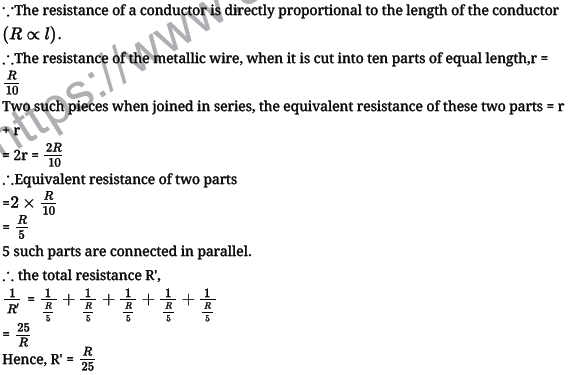Question : Which among iron and mercury a better conductor?
Answer :  Resistivity of iron is 10 × 10-8 ohm m and that of mercury 94 × 10-8 ohm m, therefore iron is a better conductor as compared to mercury.

Question : Figure shows a 240 V A.C mains circuit to which a number of appliances are connected and switched on.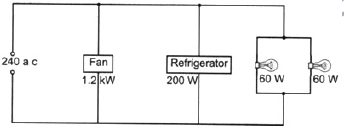i. Calculate the power supplied to the circuit.
ii. Calculate:
a. the current through the refrigerator,
b. the energy used by the fan in 3 hours,
c. the resistance of the filament of one lamp.
i. Power supplied to the circuit
= 1.2 1000 W + 200 W + 60 W + 60W
= 1200 W + 200 W + 60 W + 60 W
= 1520 W
= 1.52 kW
The power supplied to the circuit is 1.52kW
ii. a. Current in the refrigerator = Power / Voltage ( P = V x I )
= 240 W/240V
= 0.83 A
The current through the refrigerator is 0.83 A
b. Energy = Power x Time
= 1.2 kW x 3h
= 1.2 x 1000 x 3 x 60 x 60s
= 1200 x 3 x 3600 J
= 12960000 J
=  1.3 x 107
The energy used by the fan in 3 hrs is
c. Current, I = P/V
= 60/240 = 0.25 A
Resistance (Filament) = V/I
= 240/0.25= 960 Ω
The resistance of the filament of the bulb is 960

Question : A circuit diagram is given as shown below: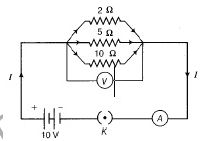Calculate
i. the total effective resistance of the circuit.
ii. the total current in the circuit.
iii. the current through each resistor.Question : Three equal resistors each equal to r and connected as shown in Fig. Calculate the equivalent resistance.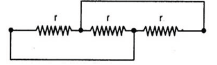Reducing the actual circuit to an equivalent circuit i.e. we find that the there resistors, each equal to r, are just placed parallel to each.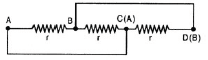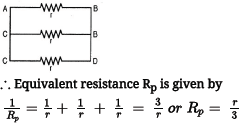Question : What is the SI unit of electric charge? How many electrons make one coulomb of charge?

Question : Define the SI unit of current.

Question : Which instrument is used to measure the current flowing in a circuit? How is it connected in the circuit and why?

Question : In which smaller units can we measure the small amount of current flowing in the circuit? How are they related to the SI unit of current?

Question : What makes the electric charge to flow?

Question : State the energy conversion taking place in

(a) Electric cell (b) Electric torch

Question : Define the electric potential difference between two points in an electric circuit carrying some current.

Question : Define the SI unit of Electric potential.

Question : Which instrument is used to measure the electric potential difference between two points in a circuit? How is it connected in the circuit and why?

Question : State the law relating the potential difference across a conductor and the current through it?

Please click the below link to access CBSE Class 10 Physics Electricity Assignment Set B